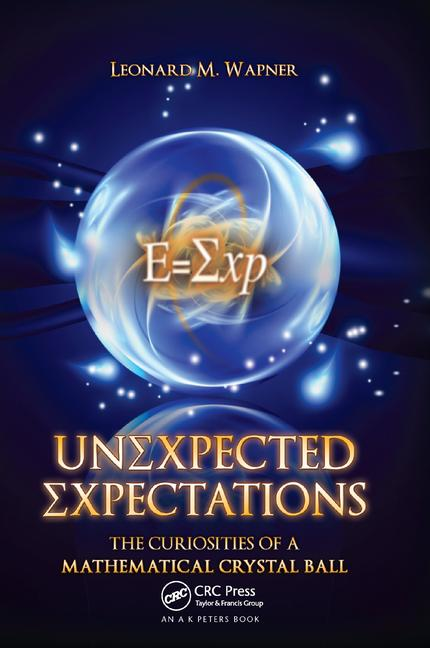# Unexpected Expectations: The Curiosities of a Mathematical Crystal Ball

## 1st Edition

Leonard M. Wapner

A K Peters/CRC Press
Published June 4, 2012
Reference - 220 Pages
ISBN 9781568817217 - CAT# K13061

For Instructors Request Inspection Copy

was \$52.95

USD\$42.36

SAVE ~\$10.59

FREE Standard Shipping!

## Preview

### Summary

Unexpected Expectations: The Curiosities of a Mathematical Crystal Ball explores how paradoxical challenges involving mathematical expectation often necessitate a reexamination of basic premises. The author takes you through mathematical paradoxes associated with seemingly straightforward applications of mathematical expectation and shows how these unexpected contradictions may push you to reconsider the legitimacy of the applications.

The book requires only an understanding of basic algebraic operations and includes supplemental mathematical background in chapter appendices. After a history of probability theory, it introduces the basic laws of probability as well as the definition and applications of mathematical expectation/expected value (E). The remainder of the text covers unexpected results related to mathematical expectation, including:

• The roles of aversion and risk in rational decision making

• A class of expected value paradoxes referred to as envelope problems

• Parrondo’s paradox—how negative (losing) expectations can be combined to give a winning result

• Problems associated with imperfect recall

• Non-zero-sum games, such as the game of chicken and the prisoner’s dilemma

• Benford’s law and its use in computer design and fraud detection

While useful in areas as diverse as game theory, quantum mechanics, and forensic science, mathematical expectation generates paradoxes that frequently leave questions unanswered yet reveal interesting surprises. Encouraging you to embrace the mysteries of mathematics, this book helps you appreciate the applications of mathematical expectation, "a statistical crystal ball."

Listen to an interview with the author on